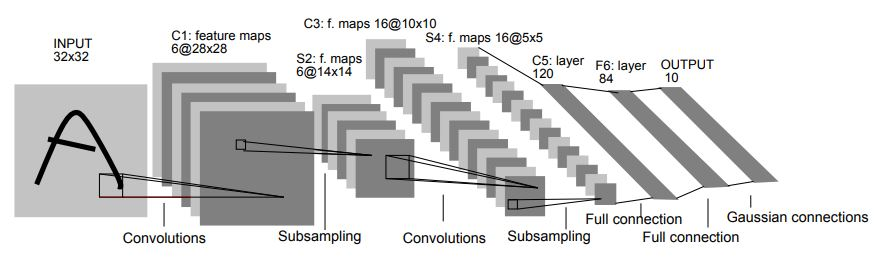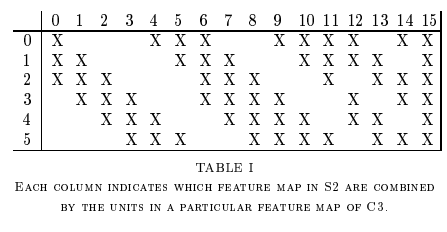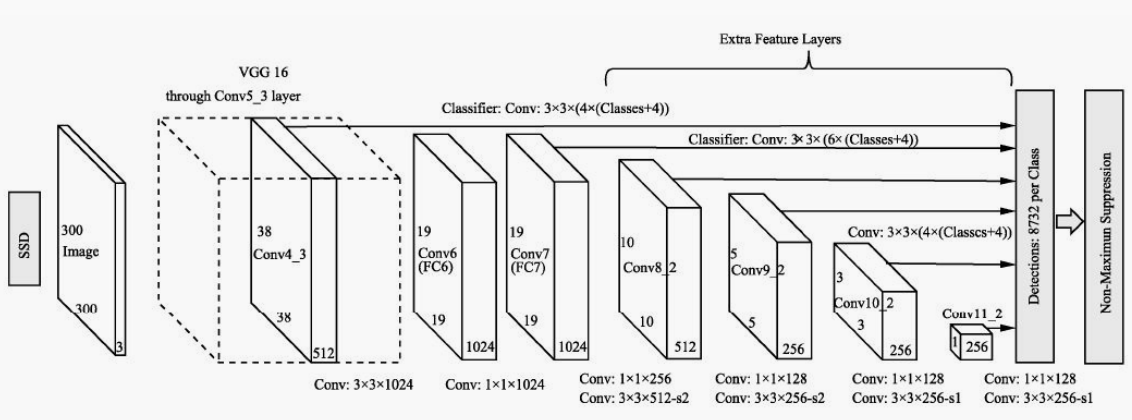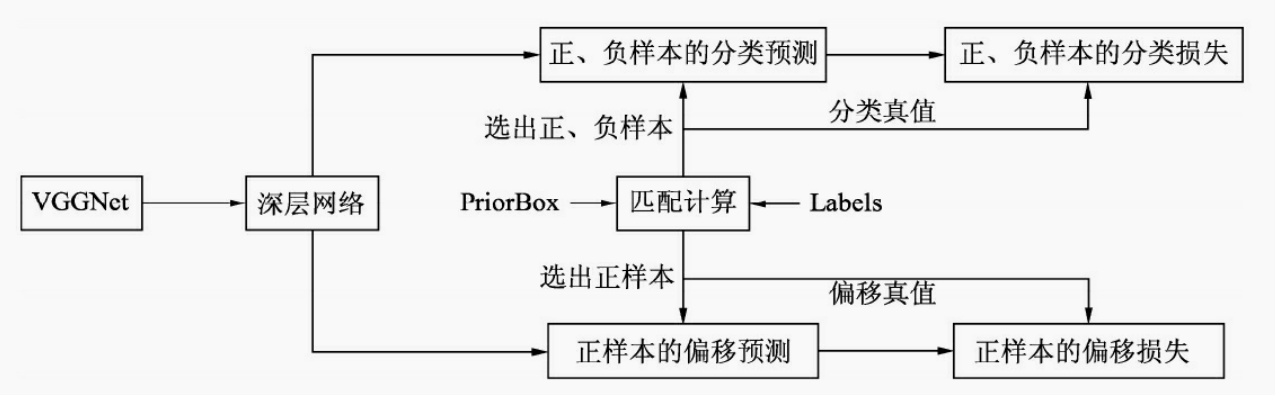• ## 简单pytorch代码流程

千次阅读 2019-04-14 20:28:49
import os os.environ['CUDA_VISIBLE_DEVICES'] = '3' os.system('echo $CUDA_VISIBLE_DEVICES') import torch import torch.nn as nn import torch.nn.functional as F import torch.utils.data as data ... import os os.environ['CUDA_VISIBLE_DEVICES'] = '3' os.system('echo$CUDA_VISIBLE_DEVICES')

import torch
import torch.nn as nn
import torch.nn.functional as F
import torch.utils.data as data
import torch.optim as optim
import numpy as np

class TransientModel(nn.Module):
def __init__(self):
super(TransientModel,self).__init__()
self.conv1 = nn.Conv2d(16, 8, kernel_size=1)
self.conv2 = nn.Conv2d(8, 4, kernel_size=1)
self.conv3 = nn.Conv2d(4, 2, kernel_size=1)
self.conv4 = nn.Conv2d(2, 1, kernel_size=1)
def forward(self, x):
x = F.relu(self.conv1(x))
x = F.relu(self.conv2(x))
x = F.relu(self.conv3(x))
x = F.relu(self.conv4(x))
return x

class MyLoss(nn.Module):
def __init__(self):
super(MyLoss, self).__init__()
print '1'
def forward(self, pred, truth):
truth = torch.mean(truth,1)
truth = truth.view(-1,2048)
pred  = pred.view(-1,2048)
return  torch.mean(torch.mean((pred-truth)**2,1),0)

class MyTrainData(data.Dataset):
def __init__(self):
self.video_path = '/data/FrameFeature/Penn/'
self.video_file = '/data/FrameFeature/Penn_train.txt'
fp = open(self.video_file,'r')
fp.close()
self.video_name = []
for line in lines:
self.video_name.append(line.strip().split(' '))
def __len__(self):
return len(self.video_name)
def __getitem__(self, index):
data = np.expand_dims(data,2)
return data

def train(model, train_loader, myloss, optimizer, epoch):
model.train()
train_data = Variable(train_data).cuda()
output = model(train_data)
loss = myloss(output, train_data)
loss.backward()
optimizer.step()
if batch_idx%100 == 0:
print('Train Epoch: {} [{}/{} ({:.0f}%)]\tloss: {:.6f}'.format(

def main():
model = TransientModel().cuda()
myloss= MyLoss()

train_data = MyTrainData()

optimizer = optim.SGD(model.parameters(),lr=0.001)

for epoch in range(10):

if __name__=='__main__':
main()


展开全文• gpu_profiling_pytorch 测试简单pytorch代码的性能 实现分布式DataParallel以在多个节点上运行 使用Nsight Profiler进行性能分析
• 二维互相关运算 mport torch import torch.nn as nn def corr2d(X, K): H, W = X.shape h, w = K.shape Y = torch.zeros(H - h + 1, W - w + 1) for i in range(Y.shape): for j in range(Y.shape): ...
• pytorch训练代码，就是这样啦，应该是实现imagenet的分类器吧
• 推荐三篇我看着很好用的博客 【1】 Pytorch预训练模型以及修改接 ... 【2】 构造一个小型CNN ...简单了解pytorch的forward https://www.cnblogs.com/ziytong/p/10677771.html
推荐三篇我看着很好用的博客
【1】 Pytorch预训练模型以及修改接 https://www.cnblogs.com/wmlj/p/9917827.html 【2】 构造一个小型CNN https://www.cnblogs.com/CATHY-MU/p/7760570.html 【3】简单了解pytorch的forward https://www.cnblogs.com/ziytong/p/10677771.html
展开全文• Pytorch代码 import torch import torch.nn as nn from torch.autograd import Variable import torch.utils.data as Data import torchvision # Hyper parameters EPOCH = 1 BATCH_SIZE = 50 LR = 0.001 DOWNLOAD_...
从零开始学习深度学习，从较早的经典模型开始学习，希望能够坚持下去，写成一个系列。LeNet5网络既经典又重要，网上关于该模型的解释数不胜数，我也就不做重复的劳动了，直接给出个人认为比较好的介绍的链接，只对其中部分内容作简要介绍，算是自己的笔记，给自己看的。
论文地址：https://ieeexplore.ieee.org/document/726791
论文详解：https://www.datalearner.com/blog/1051558664111790
模型输入： 32*32的灰度图像，每个像素的取值范围0-255。
C1层： 卷积层，6个5*5的卷积核，步长为1，得到6个28*28的图像；。
S2层： 下采样层，或者池化层，大小为2*2，平均池化，步长为2，得到6个14*14的图像。
C3层： 卷积层，16个大小为5*5的卷积核，步长为1，得到16个10*10的图像。
通常所说卷积核个数指的是后一层输出个数，但是每个卷积核中参数数量不仅是5*5，还与前一层连接数量有关。比如前一层有6张图片，经过16个卷积核，卷积核大小为5*5，输出16张图片，假设每个卷积核与前一层6张图片中每一张都进行运算，则每一个卷积核实际参数数量为：5*5*6，所有卷积核共有参数数量：5*5*6*16。
论文中，16个卷积核并不是和前一层6张图片的每一张都做卷积，而是只和前一层部分图片做卷积。其对应关系如下所是：这样卷积核的参数就由2400个，减少到了1516个。
S4层： 下采样层，或池化层，池化大小为2*2，采用平均池化，步长为2，得到16个5*5的图像。
C5层： 卷积层，120个5*5的卷积核，得到120个神经元，至此没有图像。同理，卷积核的参数数量为：5*5*16*120。
C6层： 全连接层，输出为84个神经元。
C7层： 输出层，输出10个神经元，代表数字0-9，共10类。
Pytorch代码
import torch
import torch.nn as nn
import torch.utils.data as Data
import torchvision

# Hyper parameters
EPOCH = 1
BATCH_SIZE = 50
LR = 0.001

train_data = torchvision.datasets.MNIST(
root='./mnist',
train=True,
transform=torchvision.transforms.ToTensor(),
)

#
dataset=train_data,
batch_size=BATCH_SIZE,
shuffle=True,
num_workers=2
)

test_data = torchvision.datasets.MNIST(
root='./mnist',
train=False
)
test_x = Variable(torch.unsqueeze(test_data.test_data,dim=1),volatile=True).type(torch.FloatTensor)[:2000]/255.
test_y = test_data.test_labels[:2000]

# CNN

class CNN(nn.Module):
def __init__(self):
super(CNN,self).__init__()
self.conv1 = nn.Sequential(
nn.Conv2d(       #(1*28*28)
in_channels=1,
out_channels=16,
kernel_size=5,
stride=1, #步长
),    #(16*28*28)
nn.ReLU(),
nn.MaxPool2d(kernel_size=2),#(16*14*14)
)
self.conv2 = nn.Sequential(  # 16*14*14
nn.Conv2d(16,32,5,1,2),  #32*14*14
nn.ReLU(),
nn.MaxPool2d(2)   # 32*7*7
)
self.out = nn.Linear(32*7*7,10)  #全连接

def forward(self, x):
x = self.conv1(x)
x = self.conv2(x)   #(batch,32,7,7)
x = x.view(x.size(0),-1) #(batch,32*7*7)
output = self.out(x)
return output

cnn = CNN()
# print(cnn)
loss_func = nn.CrossEntropyLoss()
# training and testing
for epoch in range(EPOCH):
b_x = Variable(x)
b_y = Variable(y)

output = cnn(b_x)
loss = loss_func(output,b_y)
loss.backward()
optimizer.step()

if step % 50 == 0:
test_output = cnn(test_x)
pred_y = torch.max(test_output,1).data.squeeze()
accuracy = sum(pred_y == test_y) / test_y.size(0)
print('Epoch: ',epoch,'| train loss: %4.f' %loss.item(),'| test accuracy: ',accuracy)

# print 10 predictions from test data
test_output = cnn(test_x[:10])
pred_y = torch.max(test_output,1).data.numpy().squeeze()
print(pred_y,'prediction number')
print(test_y[:10].numpy(),'real number')

展开全文深度学习 卷积
• 这篇博客记录我在学习《深度学习之PyTorch物体检测实战》一书中关于SSD（Single Shot Multibox Detecor）这一算法的理解，以及pytorch代码的解读。 pytorch复现代码链接：...
这篇博客记录我在学习《深度学习之PyTorch物体检测实战》一书中关于SSD（Single Shot Multibox Detecor）这一算法的理解，以及pytorch代码的解读。 pytorch复现代码链接：https://github.com/dongdonghy/Detection-PyTorch-Notebook/tree/master/chapter5/ssd-pytorch 虽然本篇博客会提到关于SSD的一些原理介绍，但是更多是侧重于pytorch代码方面的介绍，如果对SSD的原理感兴趣可以去网上搜索，有很多文章都已经介绍的很详细了。
总览
对于目标检测任务来说，一般有两种比较主流的框架，一种是Two stages，另外一种是Single shot。前者是Faster RCNN为代表，即首先利用RPN网络进行感兴趣区域生成，然后再对该区域进行类别的分类与位置的回归，这种方法优缺点很明显，虽然显著提升了精度，但也限制了检测速度。后者以以YOLO系列/SSD为代表，一次性完成classification+bounding box regression，这种利用回归的思想，虽然使速度有了明显的提升，但是精度方面也下降了不少。
下面先简单了解一下SSD的流程和算法思想。 SSD算法的算法流程如图下所示，输入图像首先经过了VGGNet的基础网络，在此之上又增加了几个卷积层，然后利用3×3的卷积核在6个大小与深浅不同的特征层上进行预测，得到预选框的分类与回归预测值，最后直接预测出结果，或者求得网络损失。整个过程中可以看出，SSD只进行了一次框的预测与损失计算，属于一阶网络。由于利用了多个特征图，SSD实现了较好的检测精度。相比于其他算法，SSD的改进可以总结为以下四点：
数据增强：SSD对原始数据做了充分的增强工作，主要包括光学变换与几何变换。网络骨架：SSD并没有直接使用VGG作为特征提取网络，而是在其卷积模块基础上，通过进一步延伸了4个卷积模块，获取不同尺度下的特征图，这些特征图具有不同的尺度与感受野，便于检测不同尺度的物体。·PriorBox与多层特征图：与Faster RCNN类似，SSD利用了固定大小与宽高的PriorBox作为区域生成，但与Faster RCNN不同的是，SSD不是只在一个特征图上设定预选框，而是在6个不同尺度上都设立预选框，并且在浅层特征图上设立较小的PriorBox来负责检测小物体，在深层特征图上设立较大的PriorBox来负责检测大物体。正、负样本的选取与损失计算：利用3×3的卷积在6个特征图上进行特征的提取，并分为分类与回归两个分支，代表所有预选框的预测值，随后进行预选框与真实框的匹配，利用IoU筛选出正样本与负样本，最终计算出分类损失与回归损失
整个计算过程如下：展开全文人工智能 深度学习
• PyTorch中“用于快速适应深度网络的与模型无关的元学习”的代码。 我重新调制了@AdrienLE IPython Notebook，使其从main.py运行，请查阅参考资料以更好地解释算法。 免责声明 我只是为了学习目的而使用它 参考Python
• 本文目的是用最简单代码，展示DQN玩游戏的效果，不涉及深度学习原理讲解。 毕竟，入门如此艰难，唯一的动力不过是看个效果，装个biu…… 安装OpenAI的游戏库gym pip install gym 看一下运行效果 import gym env ...强化学习 深度学习计算机视觉 图像处理 卷积神经网络 深度学习
• second.pytorch代码量比较大，刚开始拿到时候，我也是一头雾水，硬着头皮往下面去看，配置环境(没有跑起来的建议去下载我的docker镜像，深度学习的利器，避免二次配置软件环境问题)，让其跑起来方便调试来进行阅读。...
• 为了在 PyTorch 中计算导数，首先要创建一个张量，并设置其 requires_grad = True ，然后利用张量运算来定义函数，这里假设 u 是一个二次方的函数，而 g 是一个简单的线性函数，代码如下所示： x = torch.tensor...
• 当使用 torch.nn.DataParallel 将代码运行在多张 GPU 卡上时，PyTorch 的 BN 层默认操作是各卡上数据独立地计算均值和标准差，同步 BN 使用所有卡上的数据一起计算 BN 层的均值和标准差，缓解了当批量大小（batch ...
• 视频图matlab代码pytorch电视网 该项目包含pytorch-style的TVNet的简单pytorch实现。 简单：总共约350行代码 Pytorch样式：所有模块（中央渐变，正向渐变和散度）都扩展为torch.nn.Module。 最初的实现是在...
• 1.背景 图像到图像风格的转变是一个经典的计算机视觉问题，对于过去的图像风格迁移问题，通常都是需要一对数据，即两张图片在内容上几乎是一致的，而在风格、色彩等方面存在不同，这样的... pytorch 代码实现 待更新深度学习 神经网络 计算机视觉
• 该存储库包含U-Net和FCN的简单PyTorch实现，这是Ronneberger等人提出的深度学习细分方法。 和龙等。 用于训练的合成图像/遮罩 首先克隆存储库并cd到项目目录。 import matplotlib . pyplot as plt import numpy as...
• 代码实现 Cifar10数据集图片shape（3， 32， 32） 网络层结构： conv (3x3, 16) conv (3x3, 32) * 4 conv (3x3, 64) * 8 conv (3*3, 128) * 4 fc 10 共18层，参数量3.2M。 # 定义3x3卷积层 BatchNormal后 bias不起...深度学习 神经网络 人工智能
• ## FPN的pytorch代码实现

万次阅读 2018-09-12 15:27:53
论文地址：Feature Pyramid Networks for Object Detection 项目地址：FPN_pytorch 0x00 前言 我们在做目标检测和超分辨率重建等问题的时候，我们一般是对同一个尺...
• Attention分享 周知瑞@研发中心, Jun 20, 2018 （一）深度学习中的直觉 3 X 1 and 1 X 3 代替 3 X 3 LSTM中的门设计 生成对抗网络 Attention机制的本质来自于人类视觉注意力机制。人们视觉在感知东西的时候一般不会...Python
• 最近相关项目用到了网络模型inceptionv3及xception，并取得了不错的效果，于是相关知识整理如下(来源于网络)，并附自己对于相应网络模型的pytorch代码解析。 总共包含4个部分：inception、xception、各自代码核心...深度学习 算法
• 在当前的领域，随着需求...模型剪枝，剪枝实现起来较为简单，也是模型优化常用的方法，如果是冗余过大的模型耗时会缩减到原来的50%，下面会详细讲并分析，最后加上pytorch代码实现。 （3）.模型蒸馏，大概原理就是使用人工智能 深度学习 机器学习
• 其实整体思路挺简单的，就是将是图片拆分成很多小块，然后将小块排列成矩阵送入transformer encoder模块中计算，具体的计算过程如下图所示 我主要分享一下代码 import torch import torch.nn as nn import ...
• 一个库，可以轻松地在PyTorch中训练各种现有的GAN（生成对抗网络）。 该库主要针对GAN用户，他们希望将现有的GAN培训技术与自己的生成器/区分器一起使用。 但是，研究人员可能还会发现GAN基类对于更快地实施新的GAN...Python
• 这是我们论文的PyTorch实现： FeatMatch：基于特征的增强半监督学习，，， 2020年欧洲计算机视觉会议（ECCV） [ ] [] 抽象的 最近的最新半监督学习（SSL）方法结合使用基于图像的转换和一致性正则化作为核心组件。 ...
• ResNet结构解析及pytorch代码 标签： pytorch ResNet是恺明大神提出来的一种结构，近些年的一些结构变种，很多也是基于ResNet做的一些改进，可以说ResNet开创了更深的网络的先河，并且在很多计算机视觉学习上都取得...
• 今天小编就为大家分享一篇Pytorch实现LSTM和GRU示例，具有很好的参考价值，希望对大家有所帮助。一起跟随小编过来看看吧
• 尽管pytorch代码看起来简单而具体，但是发生的许多细微变化是不可见的，因此在使用pytorch代码时，它有助于彻底理解运行时模型。 例如，考虑以下代码： torch.nn.cross_entropy(model(images.cuda()), labels...